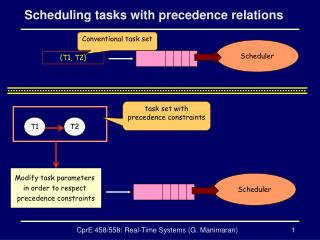Download PresentationScheduling tasks with precedence relationsScheduling tasks with precedence relations - PowerPoint PPT Presentation

Download PresentationScheduling tasks with precedence relations
An Image/Link below is provided (as is) to download presentation

Download Policy: Content on the Website is provided to you AS IS for your information and personal use and may not be sold / licensed / shared on other websites without getting consent from its author. While downloading, if for some reason you are not able to download a presentation, the publisher may have deleted the file from their server.

- - - - - - - - - - - - - - - - - - - - - - - - - - - E N D - - - - - - - - - - - - - - - - - - - - - - - - - - -
Presentation Transcript

1. Scheduling tasks with precedence relations Conventional task set Scheduler {T1, T2} task set with precedence constraints T1 T2 Modify task parameters in order to respect precedence constraints Scheduler CprE 458/558: Real-Time Systems (G. Manimaran)

2. Modifying the task parameters for RMS • While using the RMS scheduler the task parameters (ready time) need to be modified in order to respect the precedence constraints • Rj*≥ Max (Rj, Ri*) where Ri* is the modified ready time of the task Ti • Priority Prioi≥ Prioj Ti Tj CprE 458/558: Real-Time Systems (G. Manimaran)

3. Modifying ready times for RMS: example T1 1 T2 2 Initial Task Parameters T3 2 T4 1 T5 3 CprE 458/558: Real-Time Systems (G. Manimaran)

4. Modifying the Ready times for RMS R1 = 0 R2 = 5 T1 1 T2 2 R4’ = max(R1, R2,R4) R3’ = max(R1, R3) Initial Task Parameters R3 = 0 R3’ = 0 R4 = 0 T3 2 T4 1 R4’ = 5 T5 3 R5 = 0 R5’ = 5 R5’ = max(R3’, R4’,R5) CprE 458/558: Real-Time Systems (G. Manimaran)

5. Modified Ready times for RMS R1 = 0 R2 = 5 T1 1 T2 2 Modified Task Parameters R3’ = 0 T3 2 T4 1 R4’ = 5 T5 3 R5’ = 5 CprE 458/558: Real-Time Systems (G. Manimaran)

6. Assigning task priorities for RMS Assume all tasks of a connected component have the same period. Therefore, as per RMS all tasks will have a tie. We assign priorities to break the ties. R1 = 0 R2 = 5 T1 1 T2 2 R3’ = 0 T3 2 T4 1 R4’ = 5 Modified Task Parameters T5 3 R5’ = 5 CprE 458/558: Real-Time Systems (G. Manimaran)

7. Modifying task parameters for DMS • While using the DMS scheduler the task parameters (ready time, relative deadline) need to be modified in order to respect the precedence constraints • Rj*≥ Max (Rj, Ri*) • Dj*≥ Max (Dj, Di*) • Prioi≥ Prioj Ti Tj CprE 458/558: Real-Time Systems (G. Manimaran)

8. Modifying the Deadlines for DMS The procedure to modify the ready times is same as that of RMS D1 = 5 D2 = 7 T1 1 T2 2 D4’ = max(D1, D2,D4) D3’ = max(D1, D3) D3 = 5 D3’ = 5 D4 = 10 T3 2 T4 1 D4’ = 10 Modified Task Parameters T5 3 D5 = 12 D5’ = 12 D5’ = max(D3’, D4’,D5) CprE 458/558: Real-Time Systems (G. Manimaran)

9. Modifying task parameters for EDF • While using the EDF scheduler the task parameters need to be modified in order to respect the precedence constraints • Rj*≥ Max (Rj, (Ri* + Ci)) • Di*≥ Min (Di, (Dj* – Cj)) Ti Tj CprE 458/558: Real-Time Systems (G. Manimaran)

10. Modifying the Ready times for EDF R1 = 0 R2 = 5 T1 1 T2 2 R4’ = max(R1+C1, R2+C2,R4) R3’ = max(R1 + C1, R3) Initial Task Parameters R3 = 0 R3’ = 1 R4 = 0 T3 2 T4 1 R4’ = 7 T5 3 R5 = 0 R5’ = 8 R5’ = max(R3’+C3, R4’+C4,R5) CprE 458/558: Real-Time Systems (G. Manimaran)

11. Modifying the Ready times for EDF R1 = 0 R2 = 5 T1 1 T2 2 Modified Task Parameters R3’ = 1 T3 2 T4 1 R4’ = 7 T5 3 R5’ = 8 CprE 458/558: Real-Time Systems (G. Manimaran)

12. Modifying the Deadlines for EDF D2’ = Min( (D4’ – C4),(D3’ – C3), D1) D1 = 5 D2 = 7 D1’ = 3 D2’ = 7 T1 1 T2 2 D2’ = Min( (D4’ – C4), D2) Modified Task Parameters D3 = 5 T3 2 D3’ = 5 T4 1 D4 = 10 D4’ = 9 T5 3 D5 = 12 D3’ = Min( (D5 – C5), D3) D4’ = Min( (D5 – C5), D4) CprE 458/558: Real-Time Systems (G. Manimaran)

13. Modifying the Deadlines for EDF D1’ = 3 D2’ = 7 T1 1 T2 2 Modified Task Parameters T3 2 D3’ = 5 T4 1 D4’ = 9 T5 3 D5 = 12 CprE 458/558: Real-Time Systems (G. Manimaran)